Chapter 15 Thermodynamics

# 113 15.6 Entropy and the Second Law of Thermodynamics: Disorder and the Unavailability of Energy

### Summary

• Define entropy and calculate the increase of entropy in a system with reversible and irreversible processes.
• Explain the expected fate of the universe in entropic terms.
• Calculate the increasing disorder of a system.Figure 1. The ice in this drink is slowly melting. Eventually the liquid will reach thermal equilibrium, as predicted by the second law of thermodynamics. (credit: Jon Sullivan, PDPhoto.org)

There is yet another way of expressing the second law of thermodynamics. This version relates to a concept called entropy. By examining it, we shall see that the directions associated with the second law—heat transfer from hot to cold, for example—are related to the tendency in nature for systems to become disordered and for less energy to be available for use as work. The entropy of a system can in fact be shown to be a measure of its disorder and of the unavailability of energy to do work.

### MAKING CONNECTIONS: ENTROPY, ENERGY, AND WORK

Recall that the simple definition of energy is the ability to do work. Entropy is a measure of how much energy is not available to do work. Although all forms of energy are interconvertible, and all can be used to do work, it is not always possible, even in principle, to convert the entire available energy into work. That unavailable energy is of interest in thermodynamics, because the field of thermodynamics arose from efforts to convert heat to work.

We can see how entropy is defined by recalling our discussion of the Carnot engine. We noted that for a Carnot cycle, and hence for any reversible processes,$\boldsymbol{Q_{\textbf{c}}/Q_{\textbf{h}}=T_{\textbf{c}}/T_{\textbf{h}}}.$Rearranging terms yields

$\boldsymbol{\frac{Q_{\textbf{c}}}{T_{\textbf{c}}}}$$\boldsymbol{=}$$\boldsymbol{\frac{Q_{\textbf{h}}}{T_{\textbf{h}}}}$

for any reversible process.$\boldsymbol{Q_{\textbf{c}}}$and$\boldsymbol{Q_{\textbf{h}}}$are absolute values of the heat transfer at temperatures$\boldsymbol{T_{\textbf{c}}}$and$\boldsymbol{T_{\textbf{h}}},$respectively. This ratio of$\boldsymbol{Q/T}$is defined to be the change in entropy$\boldsymbol{\Delta{S}}$for a reversible process,

$\boldsymbol{\Delta{S}=}$$\boldsymbol{\left(\frac{Q}{T}\right)_{\textbf{rev}}},$

where$\boldsymbol{Q}$is the heat transfer, which is positive for heat transfer into and negative for heat transfer out of, and$\boldsymbol{T}$is the absolute temperature at which the reversible process takes place. The SI unit for entropy is joules per kelvin (J/K). If temperature changes during the process, then it is usually a good approximation (for small changes in temperature) to take$\boldsymbol{T}$to be the average temperature, avoiding the need to use integral calculus to find$\boldsymbol{\Delta{S}}.$

The definition of$\boldsymbol{\Delta{S}}$is strictly valid only for reversible processes, such as used in a Carnot engine. However, we can find$\boldsymbol{\Delta{S}}$precisely even for real, irreversible processes. The reason is that the entropy$\boldsymbol{S}$of a system, like internal energy$\boldsymbol{U},$depends only on the state of the system and not how it reached that condition. Entropy is a property of state. Thus the change in entropy$\boldsymbol{\Delta{S}}$of a system between state 1 and state 2 is the same no matter how the change occurs. We just need to find or imagine a reversible process that takes us from state 1 to state 2 and calculate$\boldsymbol{\Delta{S}}$for that process. That will be the change in entropy for any process going from state 1 to state 2. (See Figure 2.)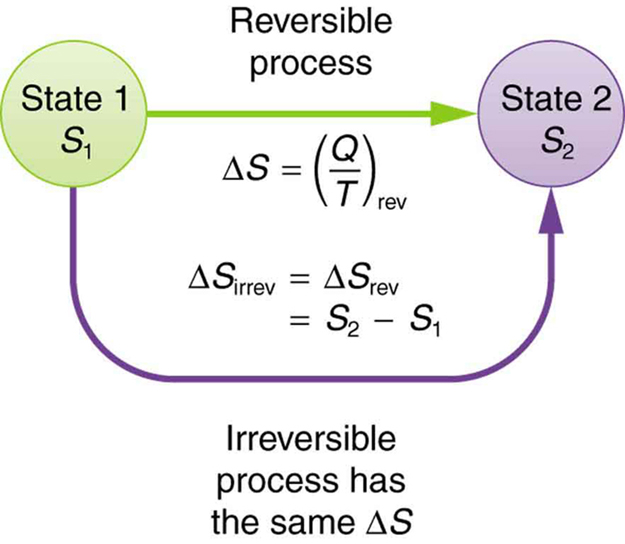Figure 2. When a system goes from state 1 to state 2, its entropy changes by the same amount ΔS, whether a hypothetical reversible path is followed or a real irreversible path is taken.

Now let us take a look at the change in entropy of a Carnot engine and its heat reservoirs for one full cycle. The hot reservoir has a loss of entropy$\boldsymbol{\Delta{S}_{\textbf{h}}=-Q_{\textbf{h}}/T_{\textbf{h}}},$because heat transfer occurs out of it (remember that when heat transfers out, then$\boldsymbol{Q}$has a negative sign). The cold reservoir has a gain of entropy$\boldsymbol{\Delta{S}_{\textbf{c}}=Q_{\textbf{c}}/T_{\textbf{c}}},$because heat transfer occurs into it. (We assume the reservoirs are sufficiently large that their temperatures are constant.) So the total change in entropy is

$\boldsymbol{\Delta{S}_{\textbf{tot}}=\Delta{S}_{\textbf{h}}+\Delta{S}_{\textbf{c}}}.$

Thus, since we know that$\boldsymbol{Q_{\textbf{h}}/T_{\textbf{h}}=Q_{\textbf{c}}/T_{\textbf{c}}}$for a Carnot engine,

$\boldsymbol{\Delta{S}_{\textbf{tot}}=-}$$\boldsymbol{\frac{Q_{\textbf{h}}}{T_{\textbf{h}}}}$$\boldsymbol{+}$$\boldsymbol{\frac{Q_{\textbf{c}}}{T_{\textbf{c}}}}$$\boldsymbol{=0}.$

This result, which has general validity, means that the total change in entropy for a system in any reversible process is zero.

The entropy of various parts of the system may change, but the total change is zero. Furthermore, the system does not affect the entropy of its surroundings, since heat transfer between them does not occur. Thus the reversible process changes neither the total entropy of the system nor the entropy of its surroundings. Sometimes this is stated as follows: Reversible processes do not affect the total entropy of the universe. Real processes are not reversible, though, and they do change total entropy. We can, however, use hypothetical reversible processes to determine the value of entropy in real, irreversible processes. The following example illustrates this point.

### Example 1: Entropy Increases in an Irreversible (Real) Process

Spontaneous heat transfer from hot to cold is an irreversible process. Calculate the total change in entropy if 4000 J of heat transfer occurs from a hot reservoir at$\boldsymbol{T_{\textbf{h}}=600\textbf{ K }(327^{\circ}\textbf{ C})}$to a cold reservoir at$\boldsymbol{T_{\textbf{c}}=250\textbf{ K }(-23^{\circ}\textbf{ C})},$assuming there is no temperature change in either reservoir. (See Figure 3.)

Strategy

How can we calculate the change in entropy for an irreversible process when$\boldsymbol{\Delta{S}_{\textbf{tot}}=\Delta{S}_{\textbf{h}}+\Delta{S}_{\textbf{c}}}$is valid only for reversible processes? Remember that the total change in entropy of the hot and cold reservoirs will be the same whether a reversible or irreversible process is involved in heat transfer from hot to cold. So we can calculate the change in entropy of the hot reservoir for a hypothetical reversible process in which 4000 J of heat transfer occurs from it; then we do the same for a hypothetical reversible process in which 4000 J of heat transfer occurs to the cold reservoir. This produces the same changes in the hot and cold reservoirs that would occur if the heat transfer were allowed to occur irreversibly between them, and so it also produces the same changes in entropy.

Solution

We now calculate the two changes in entropy using$\boldsymbol{\Delta{S}_{\textbf{tot}}=\Delta{S}_{\textbf{h}}+\Delta{S}_{\textbf{c}}}.$First, for the heat transfer from the hot reservoir,

$\boldsymbol{\Delta{S}_{\textbf{h}}=}$$\boldsymbol{\frac{-Q_{\textbf{h}}}{T_{\textbf{h}}}}$$\boldsymbol{=}$$\boldsymbol{\frac{-4000\textbf{ J}}{600\textbf{ K}}}$$\boldsymbol{=-6.67\textbf{ J/K}}.$

And for the cold reservoir,

$\boldsymbol{\Delta{S}_{\textbf{c}}=}$$\boldsymbol{\frac{Q_{\textbf{c}}}{T_{\textbf{c}}}}$$\boldsymbol{=}$$\boldsymbol{\frac{4000\textbf{ J}}{250\textbf{ K}}}$$\boldsymbol{=16.0\textbf{ J/K}}.$

Thus the total is

$\begin{array}{lcl} \boldsymbol{\Delta{S}_{\textbf{tot}}} & \boldsymbol{=} & \boldsymbol{\Delta{S}_{\textbf{h}}+\Delta{S}_{\textbf{c}}} \\ {}& \boldsymbol{=} & \boldsymbol{(-6.67 +16.0)\textbf{ J/K}} \\ {} & \boldsymbol{=} & \boldsymbol{9.33\textbf{ J/K}.} \end{array}$

Discussion

There is an increase in entropy for the system of two heat reservoirs undergoing this irreversible heat transfer. We will see that this means there is a loss of ability to do work with this transferred energy. Entropy has increased, and energy has become unavailable to do work.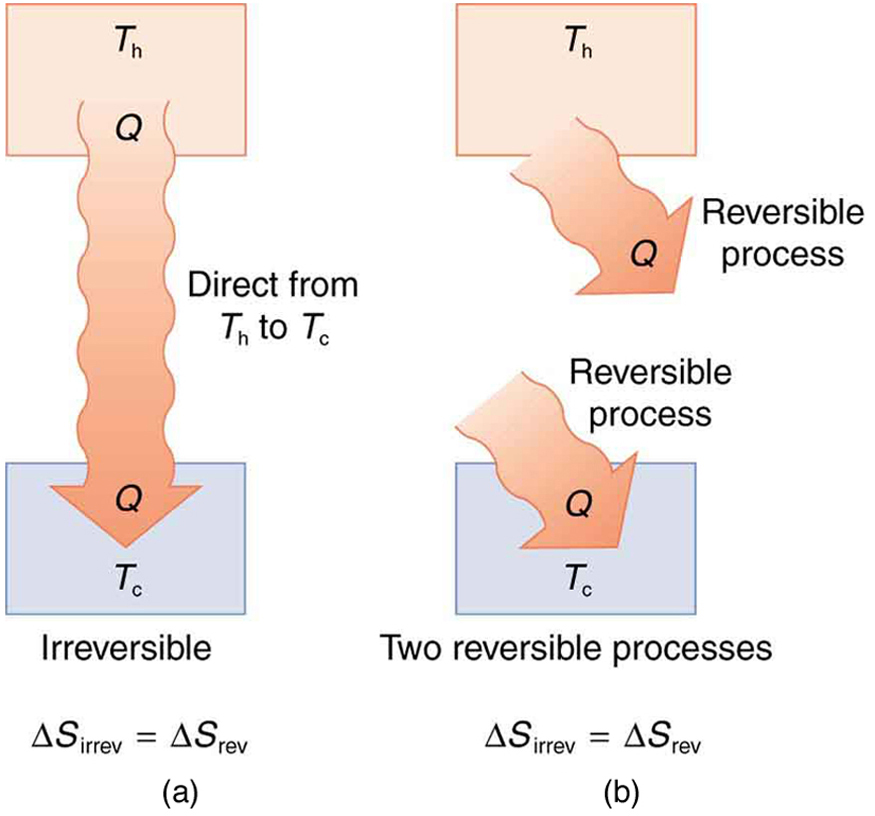Figure 3. (a) Heat transfer from a hot object to a cold one is an irreversible process that produces an overall increase in entropy. (b) The same final state and, thus, the same change in entropy is achieved for the objects if reversible heat transfer processes occur between the two objects whose temperatures are the same as the temperatures of the corresponding objects in the irreversible process.

It is reasonable that entropy increases for heat transfer from hot to cold. Since the change in entropy is$\boldsymbol{Q/T},$there is a larger change at lower temperatures. The decrease in entropy of the hot object is therefore less than the increase in entropy of the cold object, producing an overall increase, just as in the previous example. This result is very general:

There is an increase in entropy for any system undergoing an irreversible process.

With respect to entropy, there are only two possibilities: entropy is constant for a reversible process, and it increases for an irreversible process. There is a fourth version of the second law of thermodynamics stated in terms of entropy:

The total entropy of a system either increases or remains constant in any process; it never decreases.

For example, heat transfer cannot occur spontaneously from cold to hot, because entropy would decrease.

Entropy is very different from energy. Entropy is not conserved but increases in all real processes. Reversible processes (such as in Carnot engines) are the processes in which the most heat transfer to work takes place and are also the ones that keep entropy constant. Thus we are led to make a connection between entropy and the availability of energy to do work.

# Entropy and the Unavailability of Energy to Do Work

What does a change in entropy mean, and why should we be interested in it? One reason is that entropy is directly related to the fact that not all heat transfer can be converted into work. The next example gives some indication of how an increase in entropy results in less heat transfer into work.

### Example 2: Less Work is Produced by a Given Heat Transfer When Entropy Change is Greater

(a) Calculate the work output of a Carnot engine operating between temperatures of 600 K and 100 K for 4000 J of heat transfer to the engine. (b) Now suppose that the 4000 J of heat transfer occurs first from the 600 K reservoir to a 250 K reservoir (without doing any work, and this produces the increase in entropy calculated above) before transferring into a Carnot engine operating between 250 K and 100 K. What work output is produced? (See Figure 4.)

Strategy

In both parts, we must first calculate the Carnot efficiency and then the work output.

Solution (a)

The Carnot efficiency is given by

$\boldsymbol{Eff_{\textbf{C}}=1-}$$\boldsymbol{\frac{T_{\textbf{c}}}{T_{\textbf{h}}}}.$

Substituting the given temperatures yields

$\boldsymbol{Eff_{\textbf{C}}=1-}$$\boldsymbol{\frac{100\textbf{ K}}{600\textbf{ K}}}$$\boldsymbol{=0.833}.$

Now the work output can be calculated using the definition of efficiency for any heat engine as given by

$\boldsymbol{Eff=}$$\boldsymbol{\frac{W}{Q_{\textbf{h}}}}.$

Solving for$\boldsymbol{W}$and substituting known terms gives

$\begin{array}{lcl} \boldsymbol{W} & \boldsymbol{=} & \boldsymbol{Eff_{\textbf{C}}Q_{\textbf{h}}} \\ {} & \boldsymbol{=} & \boldsymbol{(0.833)(4000\textbf{ J})=3333\textbf{ J}.} \end{array}$

Solution (b)

Similarly,

$\boldsymbol{Eff^{\prime}_{\textbf{C}}=1-}$$\boldsymbol{\frac{T_{\textbf{c}}}{T^{\prime}_{\textbf{c}}}}$$\boldsymbol{=1-}$$\boldsymbol{\frac{100\textbf{ K}}{250\textbf{ K}}}$$\boldsymbol{=0.600},$

so that

$\begin{array}{lcl} \boldsymbol{W} & \boldsymbol{=} & \boldsymbol{Eff^{\prime}_{\textbf{C}}Q_{\textbf{h}}} \\ {} & \boldsymbol{=} & \boldsymbol{(0.600)(4000\textbf{ J})=2400\textbf{ J}.} \end{array}$

Discussion

There is 933 J less work from the same heat transfer in the second process. This result is important. The same heat transfer into two perfect engines produces different work outputs, because the entropy change differs in the two cases. In the second case, entropy is greater and less work is produced. Entropy is associated with the unavailability of energy to do work.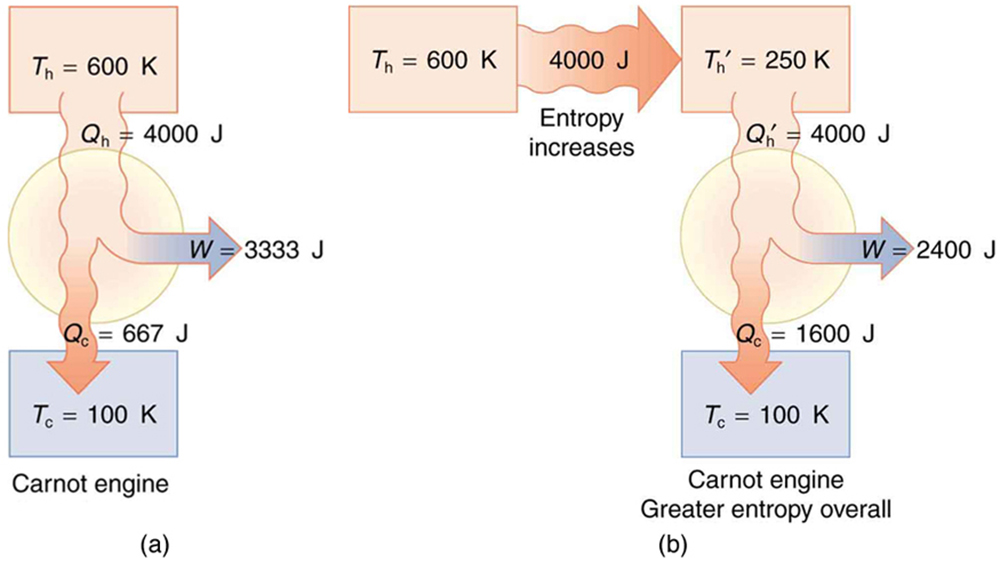Figure 4. (a) A Carnot engine working at between 600 K and 100 K has 4000 J of heat transfer and performs 3333 J of work. (b) The 4000 J of heat transfer occurs first irreversibly to a 250 K reservoir and then goes into a Carnot engine. The increase in entropy caused by the heat transfer to a colder reservoir results in a smaller work output of 2400 J. There is a permanent loss of 933 J of energy for the purpose of doing work.

When entropy increases, a certain amount of energy becomes permanently unavailable to do work. The energy is not lost, but its character is changed, so that some of it can never be converted to doing work—that is, to an organized force acting through a distance. For instance, in the previous example, 933 J less work was done after an increase in entropy of 9.33 J/K occurred in the 4000 J heat transfer from the 600 K reservoir to the 250 K reservoir. It can be shown that the amount of energy that becomes unavailable for work is

$\boldsymbol{W_{\textbf{unavail}}=\Delta{S}\cdotp{T}_0},$

where$\boldsymbol{T_0}$is the lowest temperature utilized. In the previous example,

$\boldsymbol{W_{\textbf{unavail}}=(9.33\textbf{ J/K})(100\textbf{ K})=933\textbf{ J}}$

as found.

# Heat Death of the Universe: An Overdose of Entropy

In the early, energetic universe, all matter and energy were easily interchangeable and identical in nature. Gravity played a vital role in the young universe. Although it may have seemed disorderly, and therefore, superficially entropic, in fact, there was enormous potential energy available to do work—all the future energy in the universe.

As the universe matured, temperature differences arose, which created more opportunity for work. Stars are hotter than planets, for example, which are warmer than icy asteroids, which are warmer still than the vacuum of the space between them.

Most of these are cooling down from their usually violent births, at which time they were provided with energy of their own—nuclear energy in the case of stars, volcanic energy on Earth and other planets, and so on. Without additional energy input, however, their days are numbered.

As entropy increases, less and less energy in the universe is available to do work. On Earth, we still have great stores of energy such as fossil and nuclear fuels; large-scale temperature differences, which can provide wind energy; geothermal energies due to differences in temperature in Earth’s layers; and tidal energies owing to our abundance of liquid water. As these are used, a certain fraction of the energy they contain can never be converted into doing work. Eventually, all fuels will be exhausted, all temperatures will equalize, and it will be impossible for heat engines to function, or for work to be done.

Entropy increases in a closed system, such as the universe. But in parts of the universe, for instance, in the Solar system, it is not a locally closed system. Energy flows from the Sun to the planets, replenishing Earth’s stores of energy. The Sun will continue to supply us with energy for about another five billion years. We will enjoy direct solar energy, as well as side effects of solar energy, such as wind power and biomass energy from photosynthetic plants. The energy from the Sun will keep our water at the liquid state, and the Moon’s gravitational pull will continue to provide tidal energy. But Earth’s geothermal energy will slowly run down and won’t be replenished.

But in terms of the universe, and the very long-term, very large-scale picture, the entropy of the universe is increasing, and so the availability of energy to do work is constantly decreasing. Eventually, when all stars have died, all forms of potential energy have been utilized, and all temperatures have equalized (depending on the mass of the universe, either at a very high temperature following a universal contraction, or a very low one, just before all activity ceases) there will be no possibility of doing work.

Either way, the universe is destined for thermodynamic equilibrium—maximum entropy. This is often called the heat death of the universe, and will mean the end of all activity. However, whether the universe contracts and heats up, or continues to expand and cools down, the end is not near. Calculations of black holes suggest that entropy can easily continue for at least$\boldsymbol{10^{100}}$years.

# Order to Disorder

Entropy is related not only to the unavailability of energy to do work—it is also a measure of disorder. This notion was initially postulated by Ludwig Boltzmann in the 1800s. For example, melting a block of ice means taking a highly structured and orderly system of water molecules and converting it into a disorderly liquid in which molecules have no fixed positions. (See Figure 5.) There is a large increase in entropy in the process, as seen in the following example.

### Example 3: Entropy Associated with Disorder

Find the increase in entropy of 1.00 kg of ice originally at$\boldsymbol{0^{\circ}\textbf{ C}}$that is melted to form water at$\boldsymbol{0^{\circ}\textbf{ C}}.$

Strategy

As before, the change in entropy can be calculated from the definition of$\boldsymbol{\Delta{S}}$once we find the energy$\boldsymbol{Q}$needed to melt the ice.

Solution

The change in entropy is defined as:

$\boldsymbol{\Delta{S}=}$$\boldsymbol{\frac{Q}{T}}.$

Here$\boldsymbol{Q}$is the heat transfer necessary to melt 1.00 kg of ice and is given by

$\boldsymbol{Q=mL_{\textbf{f}}},$

where$\boldsymbol{m}$is the mass and$\boldsymbol{L_{\textbf{f}}}$is the latent heat of fusion.$\boldsymbol{L_{\textbf{f}}=334\textbf{ kJ/kg}}$for water, so that

$\boldsymbol{Q=(1.00\textbf{ kg})(334\textbf{ kJ/kg})=3.34\times10^5\textbf{ J}}.$

Now the change in entropy is positive, since heat transfer occurs into the ice to cause the phase change; thus,

$\boldsymbol{\Delta{S}=}$$\boldsymbol{\frac{Q}{T}}$$\boldsymbol{=}$$\boldsymbol{\frac{3.34\times10^5\textbf{ J}}{T}}.$

$\boldsymbol{T}$is the melting temperature of ice. That is,$\boldsymbol{T=0^{\circ}\textbf{C}=273\textbf{ K}}.$So the change in entropy is

$\begin{array}{lcl} \boldsymbol{\Delta{S}} & \boldsymbol{=} & \boldsymbol{\frac{3.34\times10^5\textbf{ J}}{273\textbf{ K}}} \\ {} & \boldsymbol{=} & \boldsymbol{1.22\times10^3\textbf{ J/K}.} \end{array}$

Discussion

This is a significant increase in entropy accompanying an increase in disorder.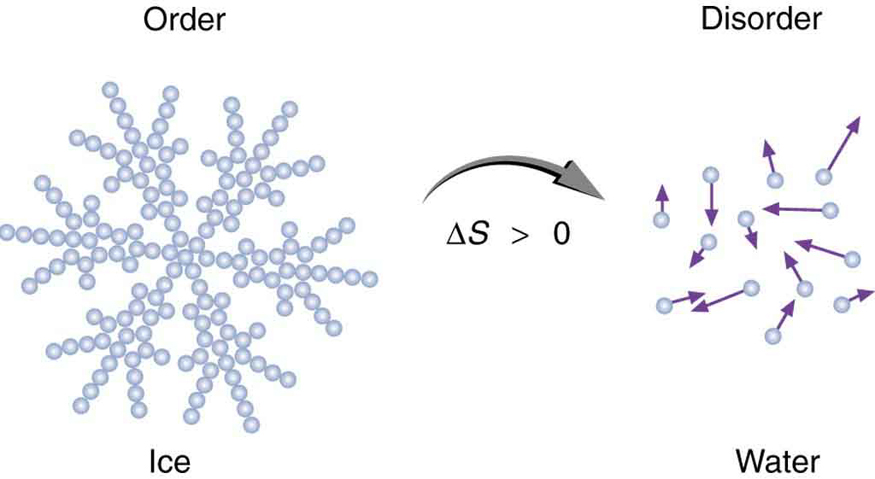Figure 5. When ice melts, it becomes more disordered and less structured. The systematic arrangement of molecules in a crystal structure is replaced by a more random and less orderly movement of molecules without fixed locations or orientations. Its entropy increases because heat transfer occurs into it. Entropy is a measure of disorder.

In another easily imagined example, suppose we mix equal masses of water originally at two different temperatures, say$\boldsymbol{20.0^{\circ}\textbf{ C}}$and$\boldsymbol{40.0^{\circ}\textbf{ C}}.$The result is water at an intermediate temperature of$\boldsymbol{30.0^{\circ}\textbf{ C}}.$Three outcomes have resulted: entropy has increased, some energy has become unavailable to do work, and the system has become less orderly. Let us think about each of these results.

First, entropy has increased for the same reason that it did in the example above. Mixing the two bodies of water has the same effect as heat transfer from the hot one and the same heat transfer into the cold one. The mixing decreases the entropy of the hot water but increases the entropy of the cold water by a greater amount, producing an overall increase in entropy.

Second, once the two masses of water are mixed, there is only one temperature—you cannot run a heat engine with them. The energy that could have been used to run a heat engine is now unavailable to do work.

Third, the mixture is less orderly, or to use another term, less structured. Rather than having two masses at different temperatures and with different distributions of molecular speeds, we now have a single mass with a uniform temperature.

These three results—entropy, unavailability of energy, and disorder—are not only related but are in fact essentially equivalent.

# Life, Evolution, and the Second Law of Thermodynamics

Some people misunderstand the second law of thermodynamics, stated in terms of entropy, to say that the process of the evolution of life violates this law. Over time, complex organisms evolved from much simpler ancestors, representing a large decrease in entropy of the Earth’s biosphere. It is a fact that living organisms have evolved to be highly structured, and much lower in entropy than the substances from which they grow. But it is always possible for the entropy of one part of the universe to decrease, provided the total change in entropy of the universe increases. In equation form, we can write this as

$\boldsymbol{\Delta{S}_{\textbf{tot}}=\Delta{S}_{\textbf{syst}}+\Delta{S}_{\textbf{envir}}>0}.$

Thus$\boldsymbol{\Delta{S}_{\textbf{syst}}}$can be negative as long as$\boldsymbol{\Delta{S}_{\textbf{envir}}}$is positive and greater in magnitude.

How is it possible for a system to decrease its entropy? Energy transfer is necessary. If I pick up marbles that are scattered about the room and put them into a cup, my work has decreased the entropy of that system. If I gather iron ore from the ground and convert it into steel and build a bridge, my work has decreased the entropy of that system. Energy coming from the Sun can decrease the entropy of local systems on Earth—that is,$\boldsymbol{\Delta{S}_{\textbf{syst}}}$is negative. But the overall entropy of the rest of the universe increases by a greater amount—that is,$\boldsymbol{\Delta{S}_{\textbf{envir}}}$is positive and greater in magnitude. Thus,$\boldsymbol{\Delta{S}_{\textbf{tot}}=\Delta{S}_{\textbf{syst}}+\Delta{S}_{\textbf{envir}}>0},$and the second law of thermodynamics is not violated.

Every time a plant stores some solar energy in the form of chemical potential energy, or an updraft of warm air lifts a soaring bird, the Earth can be viewed as a heat engine operating between a hot reservoir supplied by the Sun and a cold reservoir supplied by dark outer space—a heat engine of high complexity, causing local decreases in entropy as it uses part of the heat transfer from the Sun into deep space. There is a large total increase in entropy resulting from this massive heat transfer. A small part of this heat transfer is stored in structured systems on Earth, producing much smaller local decreases in entropy. (See Figure 6.)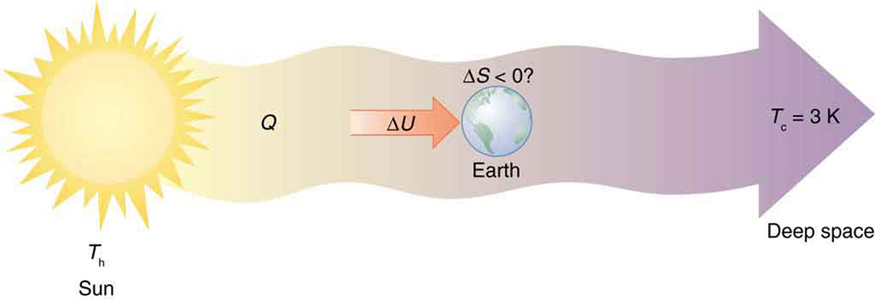Figure 6. Earth’s entropy may decrease in the process of intercepting a small part of the heat transfer from the Sun into deep space. Entropy for the entire process increases greatly while Earth becomes more structured with living systems and stored energy in various forms.

### PHET EXPLORATIONS: REVERSIBLE REACTIONS

Watch a reaction proceed over time. How does total energy affect a reaction rate? Vary temperature, barrier height, and potential energies. Record concentrations and time in order to extract rate coefficients. Do temperature dependent studies to extract Arrhenius parameters. This simulation is best used with teacher guidance because it presents an analogy of chemical reactions.# Section Summary

• Entropy is the loss of energy available to do work.
• Another form of the second law of thermodynamics states that the total entropy of a system either increases or remains constant; it never decreases.
• Entropy is zero in a reversible process; it increases in an irreversible process.
• The ultimate fate of the universe is likely to be thermodynamic equilibrium, where the universal temperature is constant and no energy is available to do work.
• Entropy is also associated with the tendency toward disorder in a closed system.

### Conceptual Questions

1: A woman shuts her summer cottage up in September and returns in June. No one has entered the cottage in the meantime. Explain what she is likely to find, in terms of the second law of thermodynamics.

2: Consider a system with a certain energy content, from which we wish to extract as much work as possible. Should the system’s entropy be high or low? Is this orderly or disorderly? Structured or uniform? Explain briefly.

3: Does a gas become more orderly when it liquefies? Does its entropy change? If so, does the entropy increase or decrease? Explain your answer.

4: Explain how water’s entropy can decrease when it freezes without violating the second law of thermodynamics. Specifically, explain what happens to the entropy of its surroundings.

5: Is a uniform-temperature gas more or less orderly than one with several different temperatures? Which is more structured? In which can heat transfer result in work done without heat transfer from another system?

6: Give an example of a spontaneous process in which a system becomes less ordered and energy becomes less available to do work. What happens to the system’s entropy in this process?

7: What is the change in entropy in an adiabatic process? Does this imply that adiabatic processes are reversible? Can a process be precisely adiabatic for a macroscopic system?

8: Does the entropy of a star increase or decrease as it radiates? Does the entropy of the space into which it radiates (which has a temperature of about 3 K) increase or decrease? What does this do to the entropy of the universe?

9: Explain why a building made of bricks has smaller entropy than the same bricks in a disorganized pile. Do this by considering the number of ways that each could be formed (the number of microstates in each macrostate).

### Problems & Exercises

1: (a) On a winter day, a certain house loses$\boldsymbol{5.00\times10^8\textbf{ J}}$of heat to the outside (about 500,000 Btu). What is the total change in entropy due to this heat transfer alone, assuming an average indoor temperature of$\boldsymbol{21.0^{\circ}\textbf{ C}}$and an average outdoor temperature of$\boldsymbol{5.00^{\circ}\textbf{ C}}?$(b) This large change in entropy implies a large amount of energy has become unavailable to do work. Where do we find more energy when such energy is lost to us?

2: On a hot summer day,$\boldsymbol{4.00\times10^6\textbf{ J}}$of heat transfer into a parked car takes place, increasing its temperature from$\boldsymbol{35.0^{\circ}\textbf{ C}}$to$\boldsymbol{45.0^{\circ}\textbf{ C}}.$What is the increase in entropy of the car due to this heat transfer alone?

3: A hot rock ejected from a volcano’s lava fountain cools from$\boldsymbol{1100^{\circ}\textbf{ C}}$to$\boldsymbol{40.0^{\circ}\textbf{ C}},$and its entropy decreases by 950 J/K. How much heat transfer occurs from the rock?

4: When$\boldsymbol{1.60\times10^5\textbf{ J}}$of heat transfer occurs into a meat pie initially at$\boldsymbol{20.0^{\circ}\textbf{ C}},$its entropy increases by 480 J/K. What is its final temperature?

5: The Sun radiates energy at the rate of$\boldsymbol{3.80\times10^{26}\textbf{ W}}$from its$\boldsymbol{5500^{\circ}\textbf{ C}}$surface into dark empty space (a negligible fraction radiates onto Earth and the other planets). The effective temperature of deep space is$\boldsymbol{-270^{\circ}\textbf{ C}}.$(a) What is the increase in entropy in one day due to this heat transfer? (b) How much work is made unavailable?

6: (a) In reaching equilibrium, how much heat transfer occurs from 1.00 kg of water at$\boldsymbol{40.0^{\circ}\textbf{ C}}$when it is placed in contact with 1.00 kg of$\boldsymbol{20.0^{\circ}\textbf{ C}}$water in reaching equilibrium? (b) What is the change in entropy due to this heat transfer? (c) How much work is made unavailable, taking the lowest temperature to be$\boldsymbol{20.0^{\circ}\textbf{ C}}?$Explicitly show how you follow the steps in the Chapter 15.7 Problem-Solving Strategies for Entropy.

7: What is the decrease in entropy of 25.0 g of water that condenses on a bathroom mirror at a temperature of$\boldsymbol{35.0^{\circ}\textbf{ C}},$assuming no change in temperature and given the latent heat of vaporization to be 2450 kJ/kg?

8: Find the increase in entropy of 1.00 kg of liquid nitrogen that starts at its boiling temperature, boils, and warms to$\boldsymbol{20.0^{\circ}\textbf{ C}}$at constant pressure.

9: A large electrical power station generates 1000 MW of electricity with an efficiency of 35.0%. (a) Calculate the heat transfer to the power station,$\boldsymbol{Q_{\textbf{h}}},$in one day. (b) How much heat transfer$\boldsymbol{Q_{\textbf{c}}}$occurs to the environment in one day? (c) If the heat transfer in the cooling towers is from$\boldsymbol{35.0^{\circ}\textbf{ C}}$water into the local air mass, which increases in temperature from$\boldsymbol{18.0^{\circ}\textbf{ C}}$to$\boldsymbol{20.0^{\circ}\textbf{ C}},$what is the total increase in entropy due to this heat transfer? (d) How much energy becomes unavailable to do work because of this increase in entropy, assuming an$\boldsymbol{18.0^{\circ}\textbf{ C}}$lowest temperature? (Part of$\boldsymbol{Q_{\textbf{c}}}$could be utilized to operate heat engines or for simply heating the surroundings, but it rarely is.)

10: (a) How much heat transfer occurs from 20.0 kg of$\boldsymbol{90.0^{\circ}\textbf{ C}}$water placed in contact with 20.0 kg of$\boldsymbol{10.0^{\circ}\textbf{ C}}$water, producing a final temperature of$\boldsymbol{50.0^{\circ}\textbf{ C}}?$(b) How much work could a Carnot engine do with this heat transfer, assuming it operates between two reservoirs at constant temperatures of$\boldsymbol{90.0^{\circ}\textbf{ C}}$and$\boldsymbol{10.0^{\circ}\textbf{ C}}?$(c) What increase in entropy is produced by mixing 20.0 kg of$\boldsymbol{90.0^{\circ}\textbf{ C}}$water with 20.0 kg of$\boldsymbol{10.0^{\circ}\textbf{ C}}$water? (d) Calculate the amount of work made unavailable by this mixing using a low temperature of$\boldsymbol{10.0^{\circ}\textbf{ C}},$and compare it with the work done by the Carnot engine. Explicitly show how you follow the steps in the Chapter 15.7 Problem-Solving Strategies for Entropy. (e) Discuss how everyday processes make increasingly more energy unavailable to do work, as implied by this problem.

## Glossary

entropy
a measurement of a system’s disorder and its inability to do work in a system
change in entropy
the ratio of heat transfer to temperature$\boldsymbol{Q/T}$
second law of thermodynamics stated in terms of entropy
the total entropy of a system either increases or remains constant; it never decreases

### Solutions

Problems & Exercises

1:

(a)$\boldsymbol{9.78\times10^4\textbf{ J/K}}$

(b) In order to gain more energy, we must generate it from things within the house, like a heat pump, human bodies, and other appliances. As you know, we use a lot of energy to keep our houses warm in the winter because of the loss of heat to the outside.

3:

$\boldsymbol{8.01\times10^5\textbf{ J}}$

5:

(a)$\boldsymbol{1.04\times10^{31}\textbf{ J/K}}$

(b)$\boldsymbol{3.28\times10^{31}\textbf{ J}}$

7:

199 J/K

9:

(a)$\boldsymbol{2.47\times10^{14}\textbf{ J}}$

(b)$\boldsymbol{1.60\times10^{14}\textbf{ J}}$

(c)$\boldsymbol{2.85\times10^{10}\textbf{ J/K}}$

(d)$\boldsymbol{8.29\times10^{12}\textbf{ J}}$

## LicenseCollege Physics: OpenStax by OpenStax is licensed under a Creative Commons Attribution 4.0 International License, except where otherwise noted.